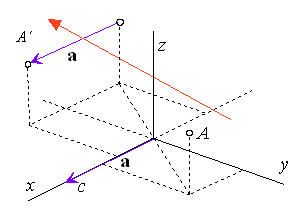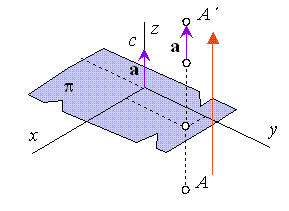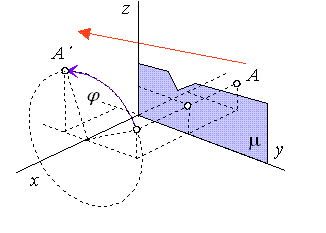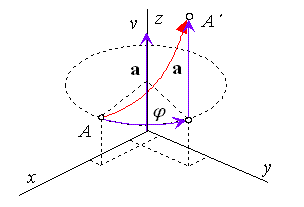# 2. Composite Euclidean transformations of space

Composite Euclidean transformations are composed from symmetries, translations and revolutions.

## 2.1 Translated symmetry about a line

Translated symmetry about a line is transformation composed from symmetry about a line and translation in the direction perpendicular to the axis of symmetry.

Let the translation vector in direction perpendicular to the axis of symmetry in the coordinate axis z be given by homogeneous coordinates $\mathbf{a}=\left(c,0,0,0\right)$, thenFigure 1: Translated symmetry about axis z

Equations and matrix of the translated symmetry about axis z

 $x\text{'}=-x+c$
 $y\text{'}=-y$
 $z\text{'}=z$
 ${\mathrm{T}}_{\mathrm{M}}=\left(\begin{array}{cccc}-1& 0& 0& 0\\ 0& -1& 0& 0\\ 0& 0& 1& 0\\ c& 0& 0& 1\end{array}\right)$

## 2.2 Translated symmetry about a plane

Translated symmetry about a plane is transformation composed from symmetry about a plane and translation in the direction perpendicular to the plane of symmetry.

Let the translation vector in direction perpendicular to the plane of symmetry in the coordinate plane $\varphi =xy$ be given by homogeneous coordinates $\mathbf{a}=\left(0,0,c,0\right)$, thenFigure 2: Translated symmetry about plane xy

Equations and matrix of the translated symmetry about plane $\varphi =xy$

 $x\text{'}=x$
 $y\text{'}=y$
 $z\text{'}=/z+c$
 ${\mathrm{T}}_{\mathrm{M}}=\left(\begin{array}{cccc}1& 0& 0& 0\\ 0& 1& 0& 0\\ 0& 0& -1& 0\\ 0& 0& c& 1\end{array}\right)$

## 2.3 Revolved symmetry about a plane

Revolved symmetry about a plane is transformation composed from symmetry about a plane and revolution about axis perpendicular to the plane of symmetry.

Let the revolved symmetry about a plane $\mu =yz$ be given by angle $\pi$ of revolution about the coordinate axis x, thenFigure 3: Revolved symmetry about plane yz

Equations and matrix of the revolved symmetry about plane $\mu =yz$

 $x\text{'}=-x$
 $y\text{'}=y\phantom{\rule{mediummathspace}{0.2em}}\mathrm{cos}\phantom{\rule{mediummathspace}{0.2em}}\pi -z\phantom{\rule{mediummathspace}{0.2em}}\mathrm{sin}\phantom{\rule{mediummathspace}{0.2em}}\pi$
 $z\text{'}=y\phantom{\rule{mediummathspace}{0.2em}}\mathrm{sin}\phantom{\rule{mediummathspace}{0.2em}}\pi +z\phantom{\rule{mediummathspace}{0.2em}}\mathrm{cos}\phantom{\rule{mediummathspace}{0.2em}}\pi$
 ${\mathrm{T}}_{\mathrm{M}}=\left(\begin{array}{cccc}-1& 0& 0& 0\\ 0& \mathrm{cos}\phantom{\rule{mediummathspace}{0.2em}}\pi & \mathrm{sin}\phantom{\rule{mediummathspace}{0.2em}}\pi & 0\\ 0& -\mathrm{sin}\phantom{\rule{mediummathspace}{0.2em}}\pi & \mathrm{cos}\phantom{\rule{mediummathspace}{0.2em}}\pi & 0\\ 0& 0& 0& 1\end{array}\right)$

## 0.4 Helical movement

Helical movement is transformation composed from revolution about a line and translation in direction of this line, axis of helical movement.

Let the translation vector in direction of coordinate axis z be given by homogeneous coordinates $\mathbf{a}=\left(0,0,v,0\right)$ and related revolution about axis z be given by the oriented angle $\pi$, thenFigure 4: Helical movement about axis z

Equations and matrix of the helical movement about axis z

 $x\text{'}=x\phantom{\rule{mediummathspace}{0.2em}}\mathrm{cos}\phantom{\rule{mediummathspace}{0.2em}}\pi -y\phantom{\rule{mediummathspace}{0.2em}}\mathrm{sin}\phantom{\rule{mediummathspace}{0.2em}}\pi$
 $y\text{'}=x\phantom{\rule{mediummathspace}{0.2em}}\mathrm{sin}\phantom{\rule{mediummathspace}{0.2em}}\pi +y\phantom{\rule{mediummathspace}{0.2em}}\mathrm{cos}\phantom{\rule{mediummathspace}{0.2em}}\pi$
 $z\text{'}=z+v$
 ${\mathrm{T}}_{\mathrm{M}}=\left(\begin{array}{cccc}\mathrm{cos}\phantom{\rule{mediummathspace}{0.2em}}\pi & \mathrm{sin}\phantom{\rule{mediummathspace}{0.2em}}\pi & 0& 0\\ -\mathrm{sin}\phantom{\rule{mediummathspace}{0.2em}}\pi & \mathrm{cos}\phantom{\rule{mediummathspace}{0.2em}}\pi & 0& 0\\ 0& 0& 1& 0\\ 0& 0& v& 1\end{array}\right)$

Trajectory of this particular helical movement is a space curve called cylindrical helix. Helix is winded on the cylinder of revolution with the axis in the axis of helical movement and basic circle in the circle of revolution of the point A. This particular helical movement is called therefore also the cylindrical helical movement.